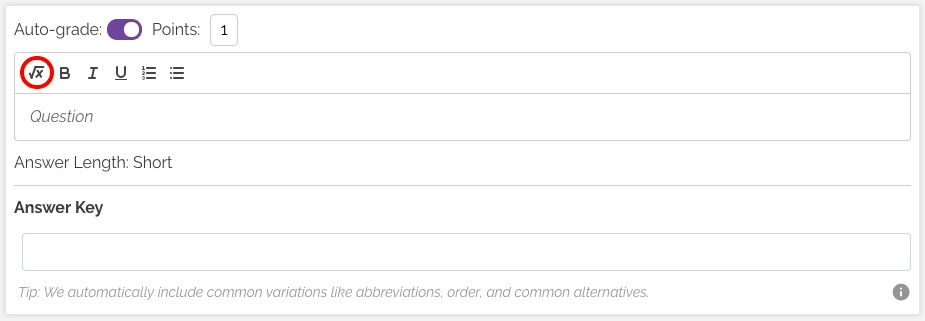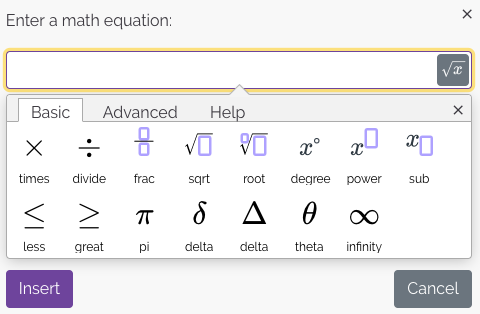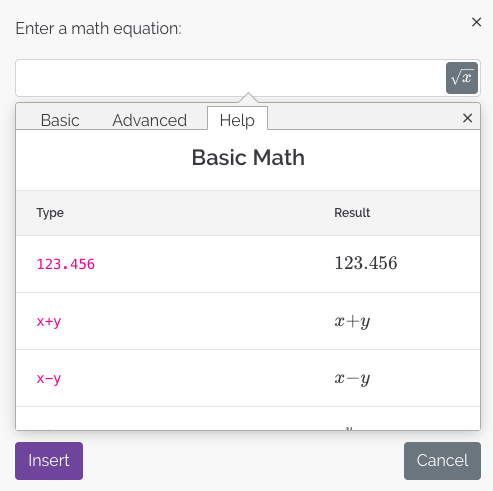# How do I type mathematical expressions?

## Q: How do I type math expressions?

Video Tutorial:

When filling in the question for a problem, click the equation icon to insert a mathematical expression.You can then select from pre-set expressions and fill in values or use the advanced setting to type your equations in LaTeX.If you need any help with writing mathematical expressions in LaTeX, the Help section will show how you can type in mathematical expressions such as fractions, exponents, integrals, symbols, and more.